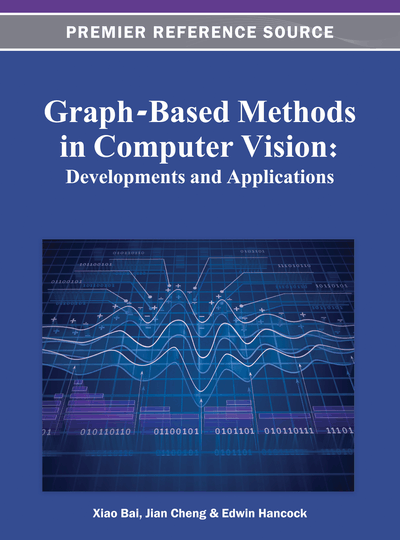# Graph Embedding Using Dissimilarities with Applications in Classification

Horst Bunke, Kaspar Riesen
DOI: 10.4018/978-1-4666-1891-6.ch008
OnDemand:
(Individual Chapters)
Available
\$29.50
No Current Special Offers

## Abstract

The domain of graphs contains only little mathematical structure. That is, most of the basic mathematical operations, actually required by many standard computer vision and pattern recognition algorithms, are not available for graphs. One of the few mathematical concepts that has been successfully transferred from the vector space to the graph domain is distance computation between graphs, commonly referred to as graph matching. Yet, distance-based pattern recognition is basically limited to nearest-neighbor classification. The present chapter reviews a novel approach for graph embedding in vector spaces built upon the concept of graph matching. The key-idea of the proposed embedding method is to use the distances of an input graph to a number of training graphs, termed prototypes, as vectorial description of the graph. That is, all graph matching procedures proposed in the literature during the last decades can be employed in this embedding framework. The rationale for such a graph embedding is to bridge the gap between the high representational power and flexibility of graphs and the large amount of algorithms available for object representations in terms of feature vectors. Hence, the proposed framework can be considered a contribution towards unifying the domains of structural and statistical pattern recognition.
Chapter Preview
Top

## Introduction

The question how to represent objects in a formal way is a key issue in the whole discipline of computer vision and pattern recognition. There are two major ways to tackle this crucial problem, viz. the statistical and the structural approach. In the statistical approach, objects are represented by feature vectors. That is, an object is formally represented as a vector x=(x1, ..., xn) ∈ Rn of n measurements. Representing objects or patterns by feature vectors x∈ Rn offers a number of useful properties, in particular, the mathematical wealth of operations available in a vector space. That is, computing the sum, the product, the mean, or the distance of two entities is well defined in vector spaces, and moreover, can be efficiently accomplished. The convenience and low computational complexity of algorithms that use feature vectors as their input have eventually resulted in a rich repository of algorithmic tools for computer vision, pattern recognition, and related fields (Duda, 2000).

However, the use of feature vectors implicates two limitations. First, as vectors always represent a predefined set of features, all vectors in a given application have to preserve the same length regardless of the size or complexity of the corresponding objects. Second, there is no direct possibility to describe binary relationships that might exist among different parts of an object. These two drawbacks are severe, particularly when the patterns under consideration are characterized by complex structural relationships rather than the statistical distribution of a fixed set of pattern features (Bunke, 1990).

The structural approach is based on symbolic data structures, such as strings, trees, or graphs, out of which graphs are the most general one. In fact, from an algorithmic perspective both strings and trees are simple instances of graphs. A string is a graph in which each node represents one symbol, and two consecutive symbols are connected by an edge. A tree is a graph in which any two nodes are connected by exactly one path. Although we focus on graphs in this chapter, the reader should keep in mind that strings and trees are always included as special cases.

The above-mentioned drawbacks of feature vectors, namely the size constraint and the lacking ability of representing relationships, can be overcome by graph based representations (Conte, 2004). In fact, graphs are not only able to describe properties of an object, but also binary relationships among different parts of the underlying object by means of edges. Note that these relationships can be of various nature (spatial, temporal, conceptual, etc.). Moreover, graphs are not constrained to a fixed size, i.e. the number of nodes and edges is not limited a priori and can be adapted to the size and the complexity of each individual object under consideration.

Yet, one drawback of graphs, when compared to feature vectors, is the significantly increased complexity of many algorithms. Nevertheless, new computer generations, which are now able to more efficiently handle complex data structures, as well as the development of fast approximate algorithms for graph comparison definitively empower researchers to use graphs in their respective problem domains (Conte, 2004). Yet, another serious limitation in the use of graphs for object classification arises from the fact that there is little mathematical structure in the domain of graphs. For example, computing the (weighted) sum or the product of a pair of entities, which are elementary operations needed in many standard algorithms in pattern recognition, is not possible in the domain of graphs, or is at least not defined in general for graph structures.

A promising approach to overcoming the lack of algorithmic tools without losing the power of graphs is offered through graph embedding in vector spaces. Basically, such an embedding of graphs establishes access to the rich repository of algorithmic tools for pattern recognition originally reserved for vectorial data. That is, graph embedding addresses the question of how to combine the complementary properties of feature vectors and graphs.

## Complete Chapter List

Search this Book:
Reset